# Boundary Extraction of image using MATLAB

• Difficulty Level : Expert
• Last Updated : 14 Mar, 2022

The boundary of the image is different from the edges in the image. Edges represent the abrupt change in pixel intensity values while the boundary of the image is the contour. As the name boundary suggests that something whose ownership changes, in the image when pixel ownership changes from one surface to another, the boundary comes into the picture. Edge is basically the boundary line but the boundary is the line or location dividing the two surfaces.

### Types of boundary extraction techniques

There are two types of boundaries in binary images.

• Inner boundary: It is the difference between the original image and the eroded image. The eroded image is the shrunk image when erosion is applied to the original image. On taking the difference between the original image and the eroded version, we get the inner boundary of the image. The inner boundary is the part of the main surface separating the other surface. Erosion shrinks the white portion thus the boundary is the part of the white surface itself.• Outer boundary: It is the difference between dilated image and an original image. The dilated image is the expanded image when dilation is applied to the original image. Dilation increases the white portion of the image. On taking the difference between dilated and original versions of the image we get the boundary which is the lost art of the black surface of the original image.### What are imerode() and imdilate()?

Erosion and Dilation both are morphological operations.

• imerode(): imerode( ) is the Matlab in-built function for erosion. imerode(I,SE) erodes the grayscale, binary, or packed binary image.

Syntax:

J = imerode(I,SE)

• imdilate( ): imdilate( ) is the MatLab in-built function for dilation.It dilates the grayscale, binary, or packed binary image I using the structuring element SE.

Syntax:

J = imdilate(I,SE)

Example 1:

## Matlab

 `% MATLAB code for INNER Boundary``% read the image.``k=imread(``"1.png"``);` `% Convert into grayscale.``k=rgb2gray(k);` `% Define structuring element.``SE=strel(``'disk'``,2,0);` `% Erode the image.``k1=imerode(k,SE);` `% Take the difference of original``% and eroded image.``k2= k-k1;` `% Display original image.``imtool(k);` `% Display inner boundary image.``imtool(k2);`

Output: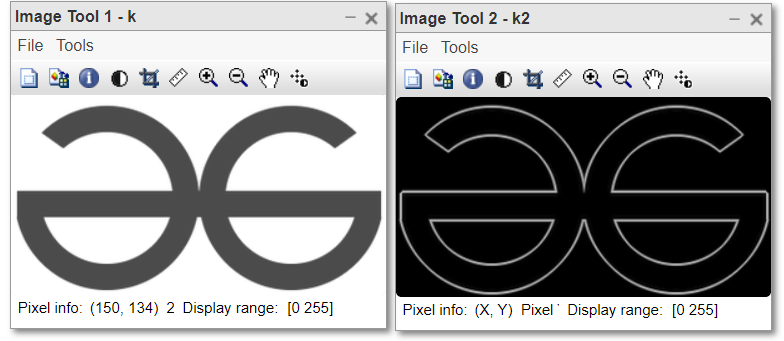Figure: Original image and Inner boundary image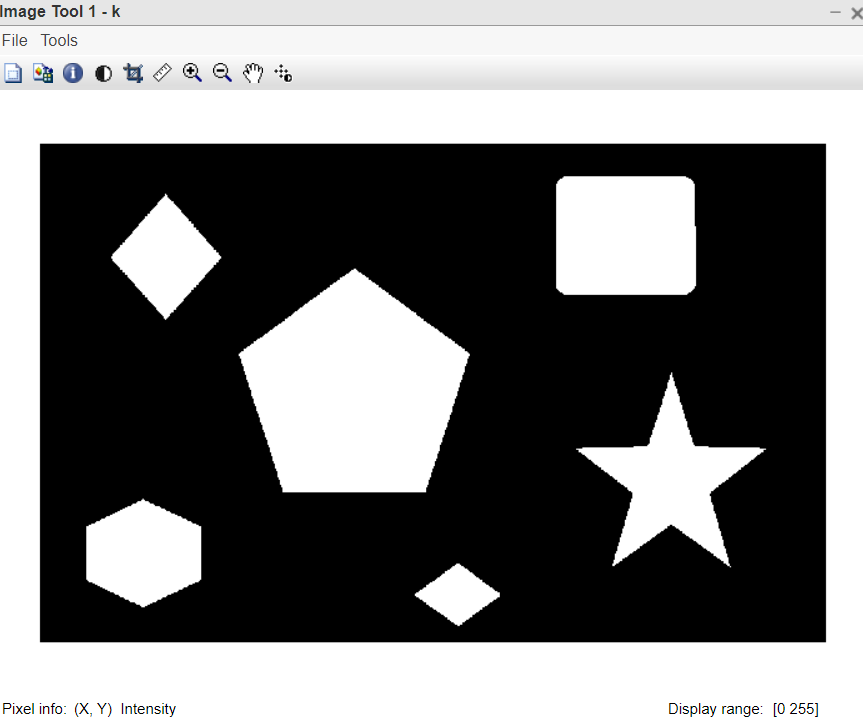figure: Original image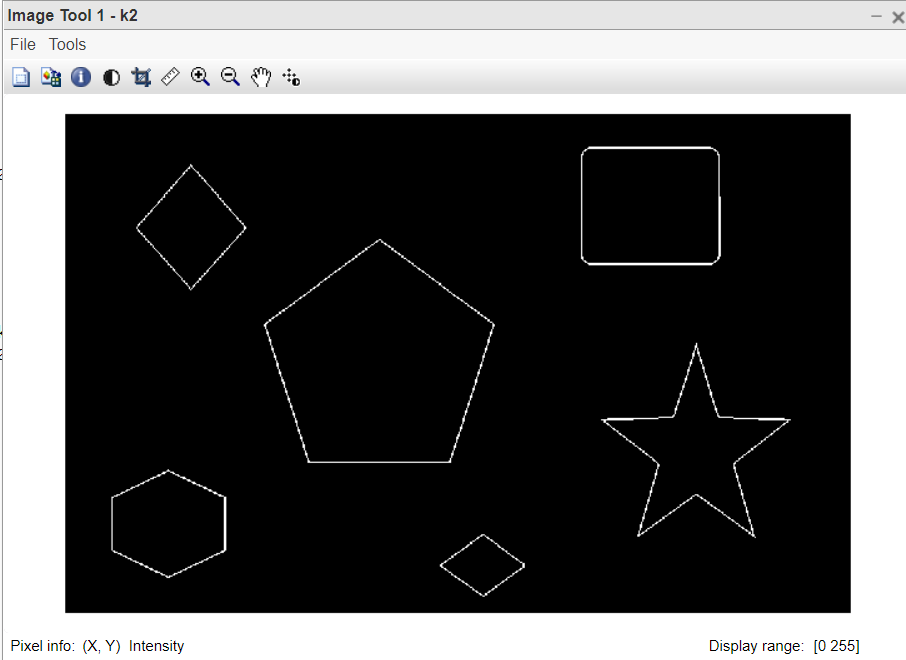figure: Inner boundary image

### Code Explanation:

• First of all, the image is read by imread( ) inbuilt function.
• Image is the colored logo of GFG, we convert it into grayscale.
• The structuring element is created for morphological operations.
• Image is eroded using imerode() inbuilt function.
• The eroded image is subtracted from the original image.
• Both images are displayed.

Example 2:

## Matlab

 `% MATLAB code OUTER Boundary``% Read the image.``k=imread(``"1.png"``);` `% Convert to grayscale.``k=rgb2gray(k);` `% Define the structuring element.``SE=strel(``'disk'``,2,0);` `% Dilate the image.``k1=imdilate(k,SE);` `% Take the difference``k2= k1-k;` `% Display original image.``imtool(k);` `% Display outer boundary image.``imtool(k2);`

Output: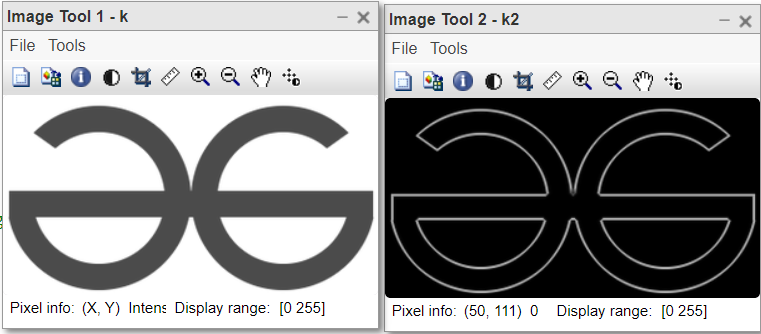figure: Original image and Outer boundary image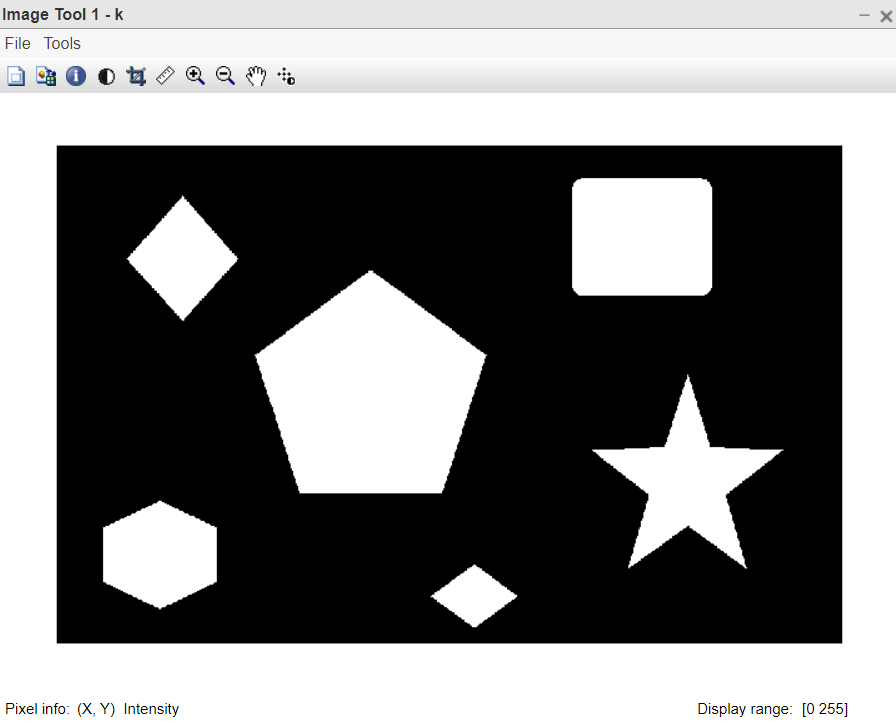figure: Original image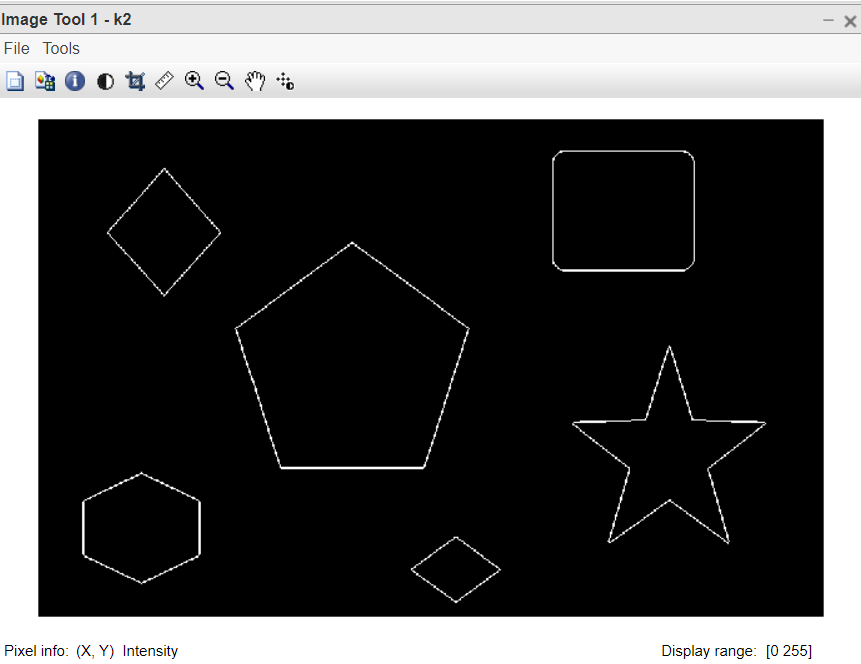figure: Outer boundary image

My Personal Notes arrow_drop_up